# Euler and Hamiltonian Paths

A graph is traversable if you can draw a path between all the vertices without retracing the same path. Based on this path, there are some categories like Euler’s path and Euler’s circuit which are described in this chapter.

## Euler’s Path

An Euler’s path contains each edge of ‘G’ exactly once and each vertex of ‘G’ at least once. A connected graph G is said to be traversable if it contains an Euler’s path.

## Example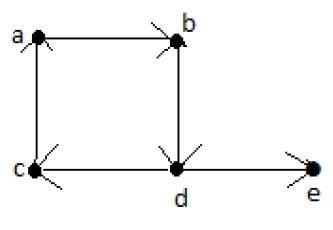Euler’s Path = d-c-a-b-d-e.

## Euler’s Circuit

In an Euler’s path, if the starting vertex is same as its ending vertex, then it is called an Euler’s circuit.

## Example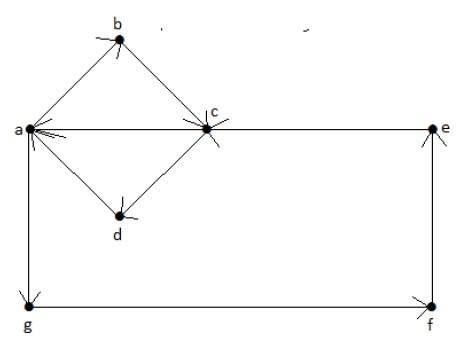Euler’s Path = a-b-c-d-a-g-f-e-c-a.

## Euler’s Circuit Theorem

A connected graph ‘G’ is traversable if and only if the number of vertices with odd degree in G is exactly 2 or 0. A connected graph G can contain an Euler’s path, but not an Euler’s circuit, if it has exactly two vertices with an odd degree.

Note − This Euler path begins with a vertex of odd degree and ends with the other vertex of odd degree.

## Example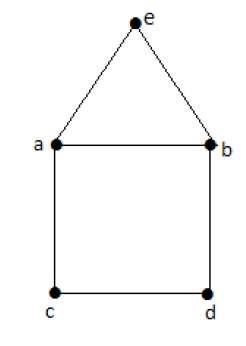Euler’s Path − b-e-a-b-d-c-a is not an Euler’s circuit, but it is an Euler’s path. Clearly it has exactly 2 odd degree vertices.

Note − In a connected graph G, if the number of vertices with odd degree = 0, then Euler’s circuit exists.

## Hamiltonian Path

A connected graph is said to be Hamiltonian if it contains each vertex of G exactly once. Such a path is called a Hamiltonian path.

## Example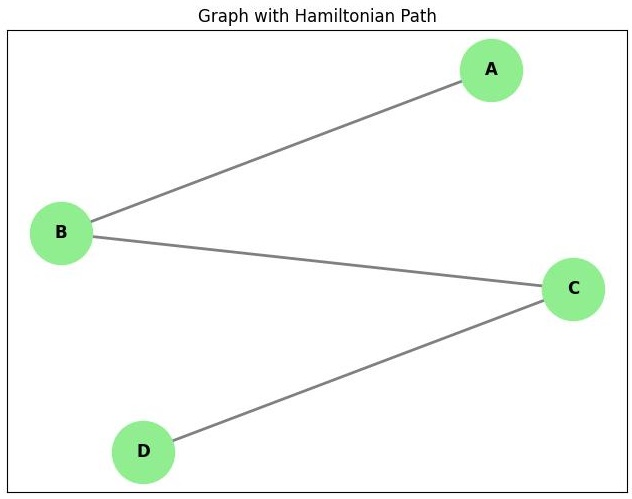Hamiltonian Path − e-d-b-a-c.

Note

• Euler’s circuit contains each edge of the graph exactly once.
• In a Hamiltonian cycle, some edges of the graph can be skipped.

## Example

Take a look at the following graph −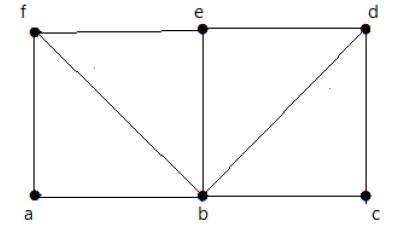For the graph shown above −

• Euler path exists – false
• Euler circuit exists – false
• Hamiltonian cycle exists – true
• Hamiltonian path exists – true

G has four vertices with odd degree, hence it is not traversable. By skipping the internal edges, the graph has a Hamiltonian cycle passing through all the vertices.